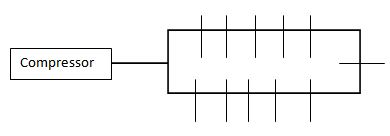## How to Size Pipes for Your Compressed Air System

Most facility’s compressed air systems have evolved over time. A spur added here a spur added there. Eventually pressure drop issues develop. Common practice is to increase the air pressure at the compressor. While it may address the symptom it does not address the problem and is very costly. For every 2 PSI increase in pressure requires 1% more energy.

A properly designed system will be a loop with spurs. This will ensure all airdrops will share the air equally. The header loop should be able to carry all the air the compressor is capable of producing.  Best practices suggest the distribution header should be sized to allow an air velocity not to exceed 30 ft/second. The formula to calculate this is:

A =    144 * Q * Pa
V *60 x (Pd +Pa)

Pipe Diameter = √ (A*4/3.14)

Where:

A = cross sectional area if the pipe bore in square inches or ∏ x diameter squared / 4
Q = Flow rate SCFM
Pa = Prevailing absolute pressure. Sea level is 14.7
Pd = compressor gauge pressure or psig.
V = Design pipe velocity ft/sec

Example: Size a header for 500 SCFM at 100 PSI at an elevation at sea level

A = 144 x 500 x 14.7 / 30 x 60 (100 + 14.7) = 5.13  square inches

Pipe diameter then is square root of  (5.13 * 4) / 3.14 = 2.56″

So an 2.56″  internal diameter pipe would be the proper size header.

The same formula can be used to calculate the sizes of the drops. In this case you would use the demand flow rate for Q.

Joe Panfalone
Application Engineer
Phone (513) 671-3322
Fax (513) 671-3363
Web: http://www.exair.com

## 11 thoughts on “How to Size Pipes for Your Compressed Air System”

1. Great little reference / reminder. Thanks

2. good reference
I don’t understand….with 144? & 60? in calculate formula..
That’s constanta or others??? Value from where
Thanks

1. Kirk Edwards says:

Azwar those conversions are the feet/inches and minutes/seconds numbers.

1. Avdhut Barve says:

Hello, do you have any calculation to interpret Energy savings in Improving the Velocity of Compressed air network?for example if netwrok has 121 m/s of velocity and we want imporve this by modfying the Pipe size to around 20 m/s than what is impact of Energy saving on Compressed air network?, please help….

3. Kirk Edwards says:

Avdhut, we would need additional information in order to provide a specific value, like your actual compressed air usage. As a general rule from the “best Practices for Compressed air” published by Compressed Air Challenge, every 2 psig reduction of pressure will produce a 1% decrease in required input power. We do assume you would increase your pipe size and deliver the same volume of air at a lower velocity and pressure.

1. Avdhut barve says:

Hello , In above formula , PD= compressor gauge pressure minus prevailing absolute pressure is this means PD for compressed air pressure 95 PSIG we need to consider PD= 95PSIG- 14.7PSIa correct?

1. Kirk Edwards says:

Yes, that is correct at sea level. This absolute pressure may change at high elevation.

2. Zak says:

what is the use of subtracting then adding 14.7 in the equation?

4. Chuy says:

Here’s a kicker that no one shows … Update this example to include the proper ACFM and pipe diameter size in Denver (5000 ft. elevation). Leave a comment when updated :)

1. Kirk Edwards says:

In this equation, the Q * Pa/(Pd + Pa) is to convert SCFM to ACFM. Another way to look at it is to look at the air compressor. Let’s say a 10HP will be de-rated in higher elevations as it will draw in less air per volume. So, at 5,000 feet, the 10 HP will act like a 8.3HP compressor. Less air means smaller pipes. This equation will work, we just need to change the actual Absolute Pressure, Pa, from 14.7 psia to 12 psia for Denver. With the example in this blog, instead of a 2.56” diameter pipe, the diameter in Denver will be 2.34” diameter.